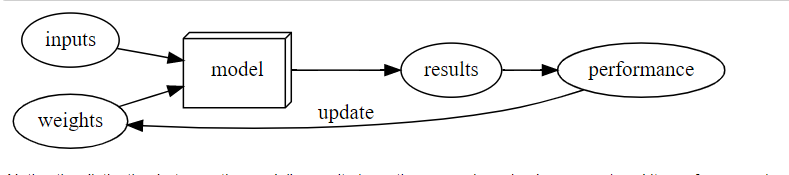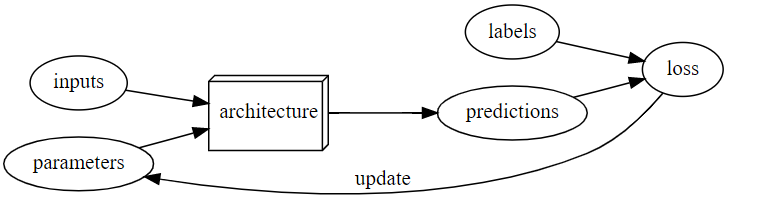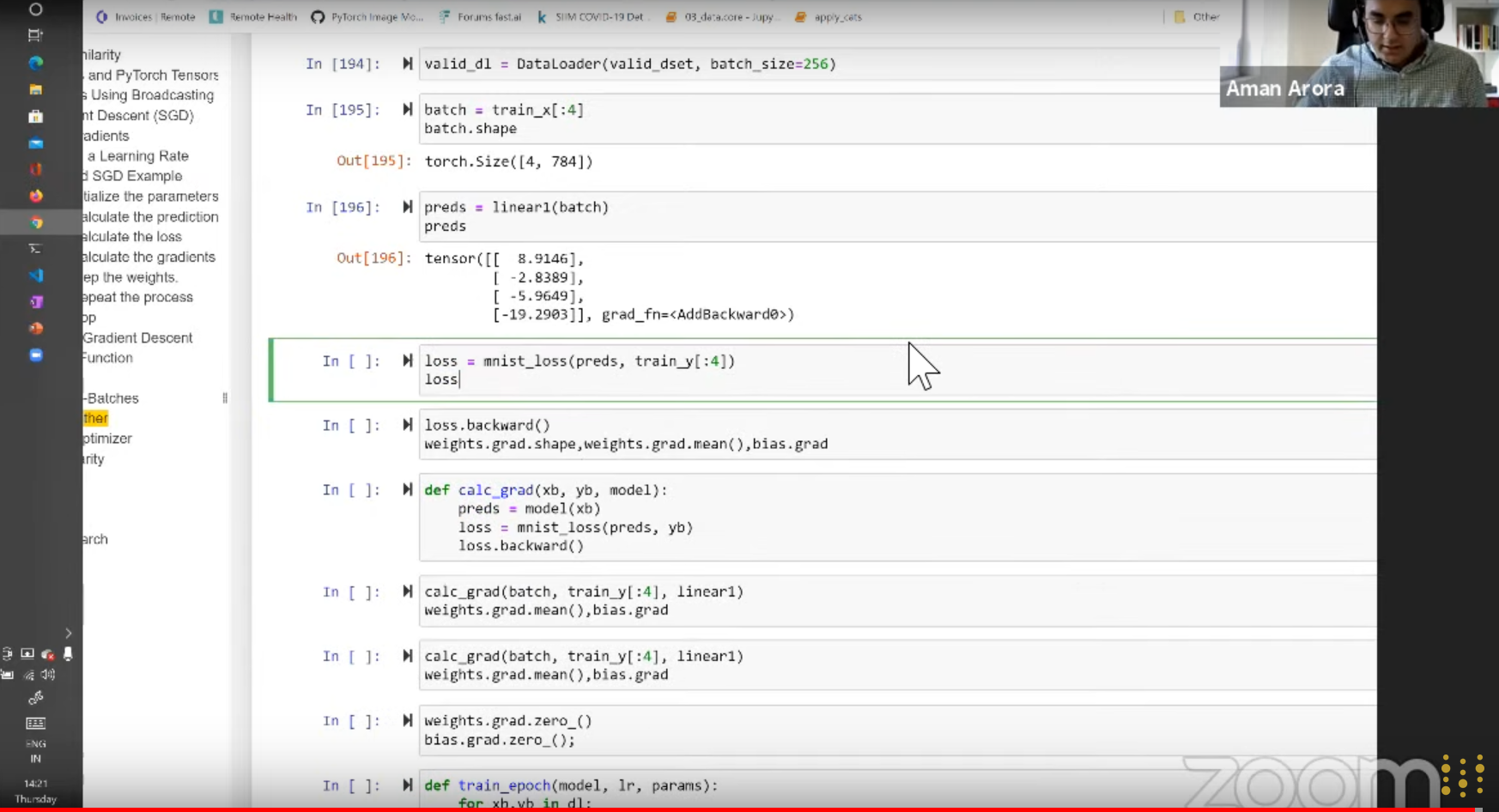Just a quick recap In the first chapter, we learned Arthur Samuel mentioned the following:

Samuel said we need an automatic means of testing the effectiveness of any current weight assignment in terms of actual performance. In the case of his checkers program, the “actual performance” of a model would be how well it plays. And you could automatically test the performance of two models by setting them to play against each other, and seeing which one usually wins. Finally, he says we need a mechanism for altering the weight assignment so as to maximize the performance. For instance, we could look at the difference in weights between the winning model and the losing model, and adjust the weights a little further in the winning direction.And the book mentions:

Neural networks - a function that is so flexible to be used to solve for any given problem SGD - a mechanism for automatically updating weight for every problem

The whole training loop of any ML task:This week in the group we were looking more into Second half of Chapter 4 which covers the gradient descent, specifically stochastic gradient descent. As a whole the week 5 was pretty code intensive and let’s looks the whole things covered this week section by section from middle of chapter 4.Since last week covered we checked how to classify 3s and 7s with pixel similarity. I was trying to classify and do all the steps using the full MNIST dataset which can be found in this Kaggle notebook.

I am sharing rough notes of each section for a better clarity, for me on what we covered in each section of Chapter 4:

It consists of seven steps:

• Initialize
• Predict
• Loss
• Step (repeat forever)
• Stop

### The MNIST Loss Function

• In Pytorch, there `view function(-1, 28*28)`, to convert a rank3-tensor(matrix) to rank-2 tensor(vectors).
• Took training and valdataion_dataset
• Used it with a model, initialized by init_params() for weights and bias which are parameters in ML model.
• We used a linear model
``````def linear1(xb):
return xb@weight + bias
``````

Then used mnist_loss() function which is calculation difference with loss and targets

### Sigmoid function

• made predictions of our function smoothen b/w vlaue 0 and 1 with sigmoid in mnist_loss()

### SGD and mini batches

DataLoader can take inputs and split inputs to small batrch to process things in GPU memory. WHole dataset need not always fit in memory

### Putting it together

-We are implementing gradient descent algorithm(7 steps here)

• Then train_epoch
• batch_accuracy
• validate_epoch

finally 96% acuracy

### Creating an optimizer

• Used Basic Optim Class maethod
• modified training_epoch function
• new train_model func()

Got 97% accuracy

### Using a non-linearity

• Use an optimizer like relu
• Use a Learner, more accruacy imporvement

In the book after this step got 98.2%

### Going Deeper

``````dls = ImageDataLoaders.from_folder(path)
learn = cnn_learner(dls, resnet18, pretrained=False,
loss_func=F.cross_entropy, metrics=accuracy)
learn.fit_one_cycle(1, 0.1)
``````

got 99% accuracy

After the lesson, I tried answering the questionnare at the end of chapter and I scored: `20/37`. I wanted to just turn up this week by writing this blogpost.

There are 2 types of people. Players and commentators. Players don’t stop playing to listen to the commentators. They just play their game.

• Prashant Choubey